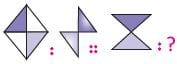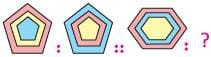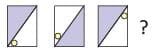Olympiad Test Level 1: Numbers’ Magic- 1

# Olympiad Test Level 1: Numbers’ Magic- 1

Test Description

## 10 Questions MCQ Test GK Olympiad for Class 3 | Olympiad Test Level 1: Numbers’ Magic- 1

Olympiad Test Level 1: Numbers’ Magic- 1 for Class 3 2023 is part of GK Olympiad for Class 3 preparation. The Olympiad Test Level 1: Numbers’ Magic- 1 questions and answers have been prepared according to the Class 3 exam syllabus.The Olympiad Test Level 1: Numbers’ Magic- 1 MCQs are made for Class 3 2023 Exam. Find important definitions, questions, notes, meanings, examples, exercises, MCQs and online tests for Olympiad Test Level 1: Numbers’ Magic- 1 below.
 1 Crore+ students have signed up on EduRev. Have you?
Olympiad Test Level 1: Numbers’ Magic- 1 - Question 1

### If yesterday was 2nd day after Wednesday, then what is today?

Detailed Solution for Olympiad Test Level 1: Numbers’ Magic- 1 - Question 1

Two days after Wednesday is Friday. If yesterday was Friday, then today is Saturday.

Olympiad Test Level 1: Numbers’ Magic- 1 - Question 2

### Identify the relation between the given pair on either side of :: and find the missing figure.Detailed Solution for Olympiad Test Level 1: Numbers’ Magic- 1 - Question 2

In the first pair of figures, the shaded portion of first figure has been removed in the second figure and the unshaded portions have been shaded. The shading has also moved by one step in clockwise direction.

Olympiad Test Level 1: Numbers’ Magic- 1 - Question 3

### Identify the relation between the given pair on either side of :: and find the missing figure.Detailed Solution for Olympiad Test Level 1: Numbers’ Magic- 1 - Question 3

In the first pair of figures, the shades in the first figure are interchanged to obtain the second figure.

Olympiad Test Level 1: Numbers’ Magic- 1 - Question 4

Which of the following figures has least number of sides?

Detailed Solution for Olympiad Test Level 1: Numbers’ Magic- 1 - Question 4

In the options, the shapes have 7, 12, 12 and 6 sides respectively.

Olympiad Test Level 1: Numbers’ Magic- 1 - Question 5

Find the odd one out.

Detailed Solution for Olympiad Test Level 1: Numbers’ Magic- 1 - Question 5

In all other figures, the shapes at both ends of the line are same.

Olympiad Test Level 1: Numbers’ Magic- 1 - Question 6

Which of the following has its weight closest to 200g?

Detailed Solution for Olympiad Test Level 1: Numbers’ Magic- 1 - Question 6

Paper is lighter than 200 g while goat and elephant are more than 200 g.

Olympiad Test Level 1: Numbers’ Magic- 1 - Question 7

How many more triangles are there than circles in the following figure?Detailed Solution for Olympiad Test Level 1: Numbers’ Magic- 1 - Question 7

The total number of triangles = 10 The total number of circles= 6 Therefore, number of triangles more than circles = 10-6= 4

Olympiad Test Level 1: Numbers’ Magic- 1 - Question 8

Which figure will replace the question mark (?) in the given figure pattern?Detailed Solution for Olympiad Test Level 1: Numbers’ Magic- 1 - Question 8

In the first pair of figures, the shaded portion given in the first figure is shifted to the other side in the second figure.

Olympiad Test Level 1: Numbers’ Magic- 1 - Question 9

Tina’s mother takes one packet of curd for Rs. 13 every day. How much money does Mini’s mother gave to the shopkeeper in the month of May for buying curd?

Detailed Solution for Olympiad Test Level 1: Numbers’ Magic- 1 - Question 9

Number of days in May = 31
Amount to be paid = Rs.13 × 31 = Rs. 403

Olympiad Test Level 1: Numbers’ Magic- 1 - Question 10

A cylinder has

Detailed Solution for Olympiad Test Level 1: Numbers’ Magic- 1 - Question 10

A cylinder is a threedimensional shape with 3 faces, 2 edges and no vertex.

## GK Olympiad for Class 3

48 tests
 Use Code STAYHOME200 and get INR 200 additional OFF Use Coupon Code